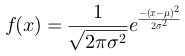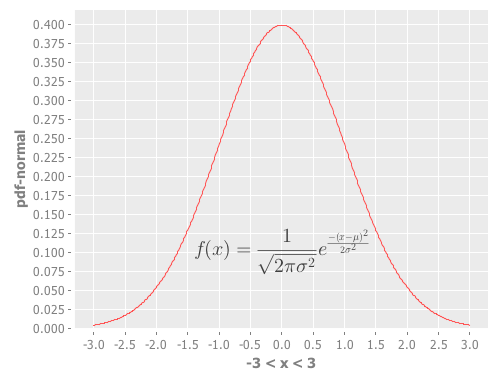# Category Archives: LaTeX

## Adding LaTeX equations to Incanter charts

I’ve added a new library to Incanter called incanter.latex that adds the ability to include LaTeX formatted equations as annotations and subtitles in charts. The library is based on the fantastically useful JLaTeXMath library.

The following examples require Incanter version 1.2.2-SNAPSHOT or greater. Add the following dependency to your project.clj file:

[incanter "1.2.2-SNAPSHOT"]


(use '(incanter core stats charts latex))


Define the latex-formatted equation; I’ll use the str function so I can break the equation across multiple lines. Notice that I have to use two backslashes where I would only need one if I were were working directly in LaTeX; this is because the backslash is an escape character in Clojure/Java strings.

(def eq (str "f(x)=\\frac{1}{\\sqrt{2\\pi \\sigma^2}}"
"e^{\\frac{-(x - \\mu)^2}{2 \\sigma^2}}"))


The equation can be rendered as an image with the latex function. The rendered equation can then be viewed in a window or saved as a png file with the view and save functions respectively.

(view (latex eq))
(save (latex eq) filename)Use the add-latex function to add an annotation to a chart. The following example adds the above equation to a function-plot of the Normal PDF.

(doto (function-plot pdf-normal -3 3)
view)Use the add-latex-subtitle function to add a rendered LaTeX equation as a subtitle to the chart (this particular chart does not have a main title).

(doto (function-plot pdf-normal -3 3)